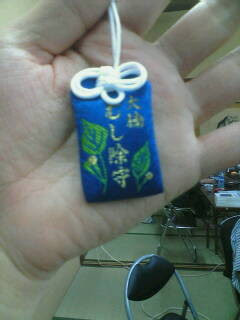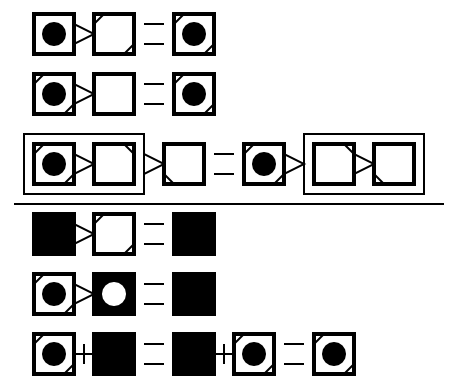## Saturday, April 18, 2009

### bug repellent talisman

Hi, it is NISHIO Hirokazu. I'm attending Hackathon with Spa and monjudoh found a talisman of bug repellent at nearby shrine.Originally the talisman is for farmers, but of course, we also suffer from bugs. It works! :-)

## Tuesday, April 14, 2009

### Can you see what the diagram means?

Hi, it's NISHIO Hirokazu. Today I translate one of my 'quiz' entry. It is for programmers who know a functional programming language.

See following image.

• What do "black-centered squares" mean?
• What do "white-centered squares" mean?
• What do ">"-like shape mean?
• What do "+"-like shape mean?If you have learned Haskell you should have been confused by those rules.

• The first 3 lines mean monad laws.
`1. (return x) >>= f == f x2. m >>= return == m3. (m >>= f) >>= g == m >>= (\x -> f x >>= g)`

• White-centered squares --- if you put a black circle in them, they become black-centered squares --- mean functions which take something as argument and return a monad. (Monad m) => a -> m b
• Fully white squares mean return :: (Monad m) => a -> m a
• ">"-like shapes mean (>>=) :: (Monad m) => m a -> (a -> m b) -> m b.
It have a black-centered square(monad) on its left-side and a white-centered square(function) on its right side.

You see ">"-like shape on the third line have a white-centered square on its left. A black circle x was removed from a monad (f x >>= g). So it means (\x -> f x >>= g). It seem like (f >>= g) but it cause type error.

It is a little unclear because it is not symmetrical. But if you remove all black circles, those have same structure as 1 * x = x, x * 1 = x, (x * y) * z = x * (y * z)

Now let's see the last 3 lines.

• The last 3 lines mean MonadPlus's law
`1. mzero >>= f == mzero2. m >>= (\x -> mzero) == mzero3. mzero `mplus` m == m `mplus` mzero == m`

• Fully black square means mzero.
• "+"-like shape means mplus.
• A black square with white hole, in the other word "A fully black square which was removed a black circle", means (\x -> mzero)

If you remove all black circles again, you'll see these 3 lines have same structure as 0 * x = 0, x * 0 = 0, x + 0 = 0 + x = x.

This figure is very beautiful because it shows mathematical structure behind haskell.